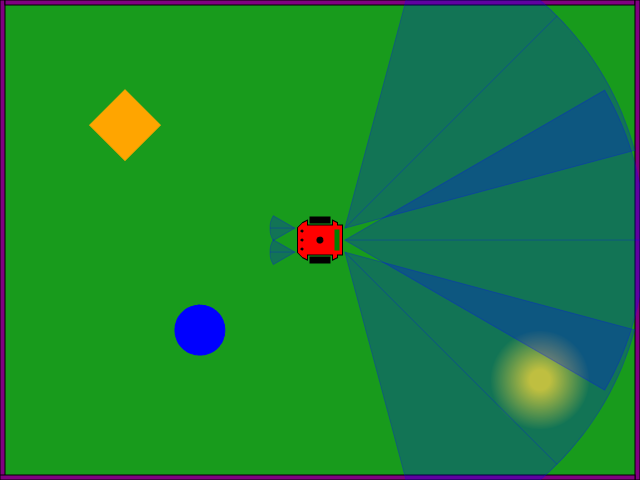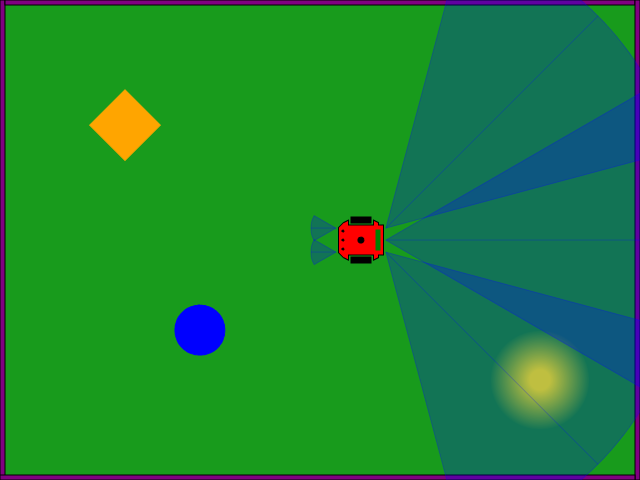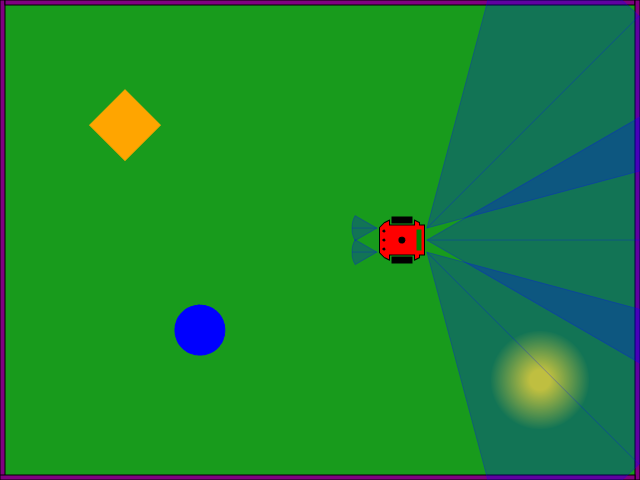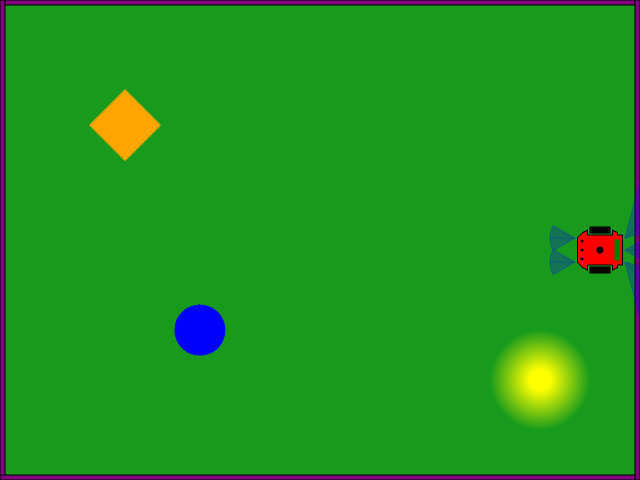# Can a robot do something that it wasn't trained to do?¶

Depends on what we mean! If we mean: "can a robot do something that it wasn't exactly trained to do?", then the answer is "of course!" because, if controlled by a neural network, we know that a NN can generalize (interpolate).

If we mean: "can a robot extrapolate?" then we don't know. Extrapolation means to be able to go beyond what it was trained to do.

Let's do a simple robot learning experiment to explore this question. First, let's start up a real robot or a simulated one:

In :
import Myro
Myro.init("sim")
robot = Myro.getRobot()
Myro.setOption("show-sensors", True)

You are using:
Simulated Fluke, version 1.0.0
Simulated Scribbler 2, version 1.0.0
Hello, my name is 'Scribby'!


That should bring up a window like the following:

In :
Myro.getSimulation()

Out:The infrared sensors (transparent blue pie pieces) work by hitting an obstacle, and bouncing back to the robot. The three on the front of the robot are much more sensitive than the ones on the back. The center-front sensor can be read by issuing robot.getObstacle(1):

In :
robot.getObstacle(1)

Out:
0

The robot is too far from the wall. Let's move the robot forward at fullspeed (1), for one second (1). The command for that is robot.forward(POWER, TIME):

In :
robot.forward(1, 1)


That will put the robot about right here (your milleage will vary... there is variation in the robot control and communication):

In :
Myro.getSimulation()

Out:Now we check the reading again:

In :
robot.getObstacle(1)

Out:
14.37485

Move a bit closer, and check again:

In :
robot.forward(1,1)
print(robot.getObstacle(1))

700.982

In :
Myro.getSimulation()

Out:Interesting. The sensor reading gets larger the closer the robot gets to the wall. If we did further testing we would also find the up close, the reading is about 6400, and varies in a non-linear manner in relation to the distance.

We will use this single sensor in an attempt to let a neural network drive the robot. First, we will design a teacher program that will determine what the speed of the robot should be. As the robot gets closer to the wall, the teacher will instruct the robot to change speeds, using the robot.forward(POWER) command.

We need to create a neural network for the training.

We create a 1 input, 3 hidden, 1 output neural network as described in Neural Networks. In this example, we use a GovernorNetwork which helps with Catastrophic Forgetting.

In :
from ai.governor import GovernorNetwork
net = GovernorNetwork(2, 0.1, 0.1) # size of inputs + outputs
trial_count = 0
count = 0

Conx using seed: 1398396134.70


The following function is the teacher. It is very easy to capture the desired output as "rules":

In :
def teacher(distance):
if distance < .5:
target = 0.5
elif distance < .8:
target = .66
elif distance < .99:
target = .83
else:
target = 1.0
return target


The goal here is to let a NN watch a teacher drive the robot.

After training, we can remove the teacher, and see how well the NN can drive the robot.

Now, let's start the training:

In :
robot.setPose(100, 250, 0)
net.setLearning(1)
t = 0

while True:
count += 1
obstacle = robot.getObstacle(1)
distance = float(1 - obstacle/6400)
target = teacher(distance)
speed = target * 2 - 1.0
if distance < .5:
if t == 0:
robot.stop()
t = Myro.currentTime()
elif Myro.currentTime() - t >= 1.5:
trial_count += 1
robot.setPose(100, 250, 0)
t = 0
# else keep waiting
else:
robot.forward(speed)
results = net.step(input=[distance], output=[target])
calico.animate("Trails: %s, Updates: %s" % (trial_count, count), str(net))

'Trails: 18, Updates: 2429'
'Layer 'output': (Kind: Output, Size: 1, Active: 1, Frozen: 0)
Target    : 1.00
Activation: 0.94
Layer 'hidden': (Kind: Hidden, Size: 3, Active: 1, Frozen: 0)
Activation: 0.72  0.68  0.70
Layer 'input': (Kind: Input, Size: 1, Active: 1, Frozen: 0)
Activation: 1.00
'
Running script aborted!


And let's test to see how well the neural network has learned what the teacher is doing.

First, turn learning off, we position the robot, and initialize some variables so we can collect and analyze the results.

In :
net.setLearning(0)
robot.setPose(100, 250, 0)
data = []
steps = []
step = 0


Let's see what the network says to do in each of the positions:

In :
while True:
obstacle = robot.getObstacle(1)
distance = float(1 - obstacle/6400)
results = net.propagate(input=[distance])
data.append([distance, teacher(distance), results])
steps.append([step, teacher(distance), results])
speed = (results * 2) - 1.0
robot.forward(speed)
calico.animate(speed)
step += 1

0.0233274382577215
Running script aborted!


Let's plot the teacher vs the network:

In :
calico.ScatterChart(['step', 'Teacher', 'Network'], steps,
{"hAxis": {"title": "step"}, "vAxis": {"title": "output"}, "height": 500})

Out:

And let's also see the data as it was learned, mapping a given input (horizontal) to the desired output (vertical).

In :
calico.ScatterChart(['distance', 'Teacher', 'Network'], data,
{"hAxis": {"title": "distance"}, "vAxis": {"title": "output"}, "height": 500})

Out:

## Results¶

• Network generalizes, but doesn't capture "step function"... yet
• Could train more, and add more hidden units, to better capture the function
• Learns the full range, from nearly full speed to stop

But, what does it do when it is put outside of its training data? Can it extrapolate?

After learning well enough, let's put the robot in a position it has never been in before:

In :
robot.setPose(600, 250, 0)

In :
Myro.getSimulation()

Out:In :
data_gen = []

while True:
obstacle = robot.getObstacle(1)
distance = float(1 - obstacle/6400)
results = net.propagate(input=[distance])
data_gen.append([distance, None, results])
speed = (results * 2) - 1.0
robot.forward(speed)
calico.animate(speed)

-0.000307838272625371
Running script aborted!


What does the robot do? How can you explain that?

In :
calico.ScatterChart(['distance', 'Teacher', 'Network'], data + data_gen,
{"hAxis": {"title": "distance"}, "vAxis": {"title": "output"}, "height": 500})

Out:

But what does this mean to the robot?

# Lessons Learned¶

• NN Learning can interpolate and extrapolate
• Extrapolation and interpolation are not necessarily different things internally;
• it depends on representation
• A system doesn't necessarily think of a problem in the way that we verbalize it
• We don't necessarily think the way that we think we do
In [ ]: